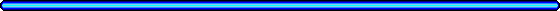# Math Tricksprepared by
Dr. Myron Hlynka, Dept. of Math. & Stat, U. of Windsor.
May 12, 2012.

## May 12, 2012 Science Rendezvous Mathematics and Probability Tricks.

1. The Vanishing Leprechaun. Shift two pieces of paper and the count of the number of leprechauns changes.
How is this possible?
(Category: geometry)
2. Stack up 5 dice randomly and the mathemagician will sum the numbers hidden in the centre column?
How can he do this?
3. Pick a 3 digit integer and rearrange the digits to get a second 3 digit integer.
Subtract the smaller number from the larger number.
The mathemagician will ask for all but one digit and then predict the missing digit.
Amazing!!
(Category: number theory, congruence)
4. Choose a 2 digit number that is not divisible by 3.
Raise the number to the 6th power and tell the mathemagician all of the digits except one.
The mathemagician will announce the missing digit.
(Category: number theory, Euler's Theorem)
5. Throw a die until it settles on a number like (2,3,4,5,6).
The mathemagician has a sealed envelope prepared to determine the number on which you stopped.
(Category: number theory, probability)
6. A set of dice are arranged in a rectangular chocolate box. Pick a starting value and count your way until the end of the box.
Without knowing your start value, the mathemagician will determine your end value.
(Category: probability, stochastic coupling)
7. A deck of cards is sorted so that red and black cards alternate. The deck is then shuffled exactly once with a riffle shuffle.
After shuffling, the cards are removed in pairs. What is the probability that each pair contains one red and one black card?
It's not what you might think! (Category: probability, proof by induction)
8. Sicherman dice. New for 2012 Science Rendezous. !!.
Your mathemagician is Dr. Myron Hlynka.
http://web2.uwindsor.ca/math/hlynka/index.html